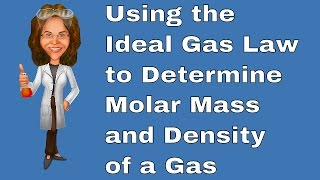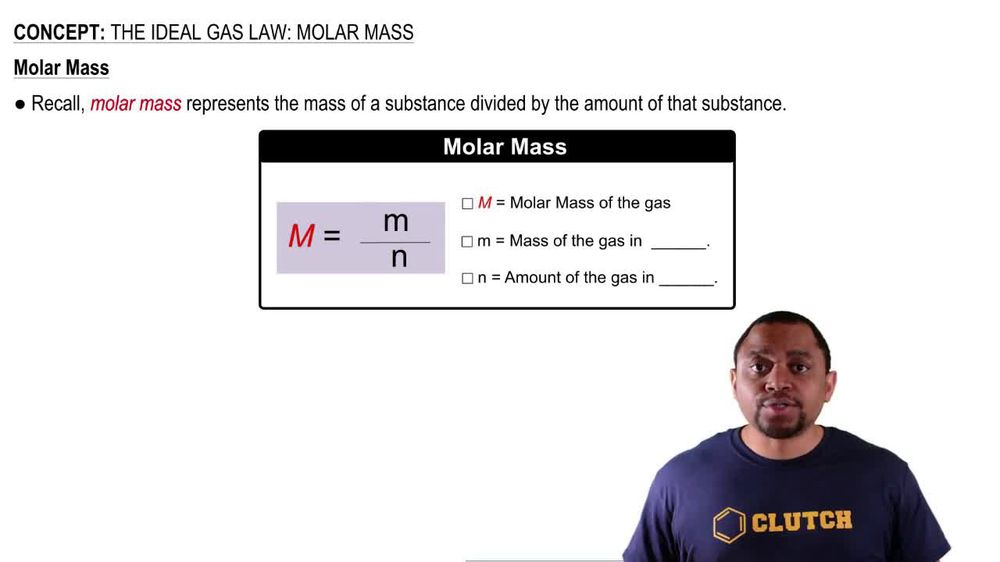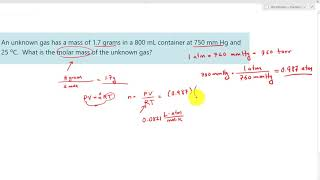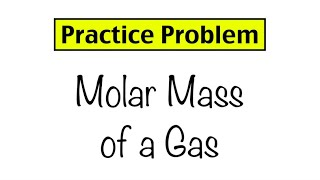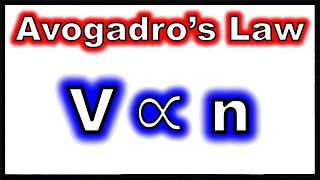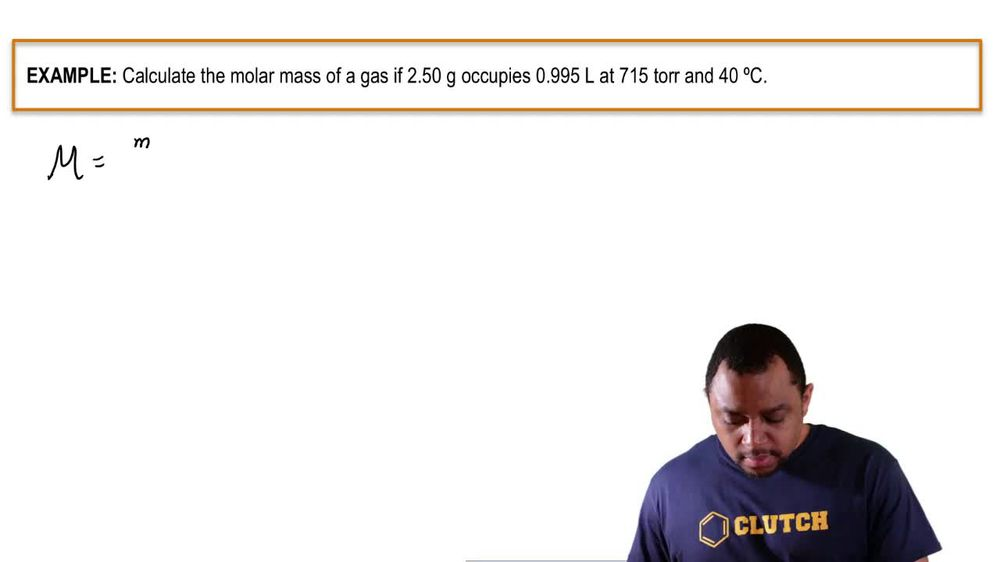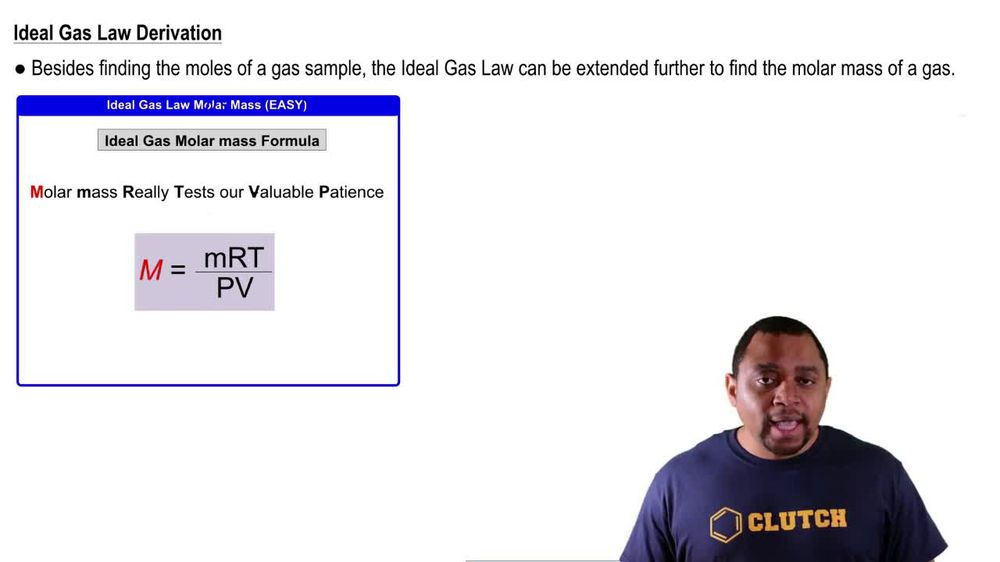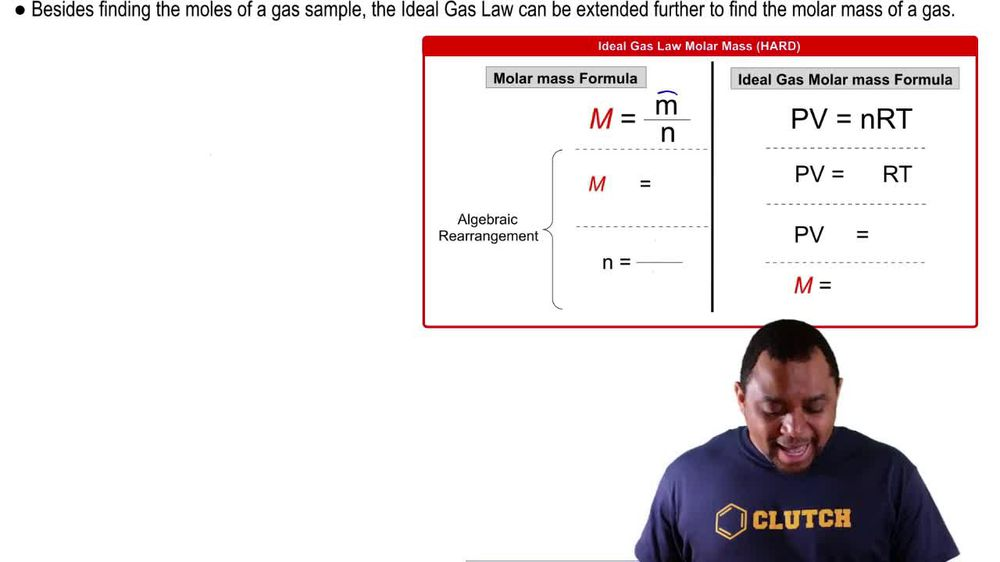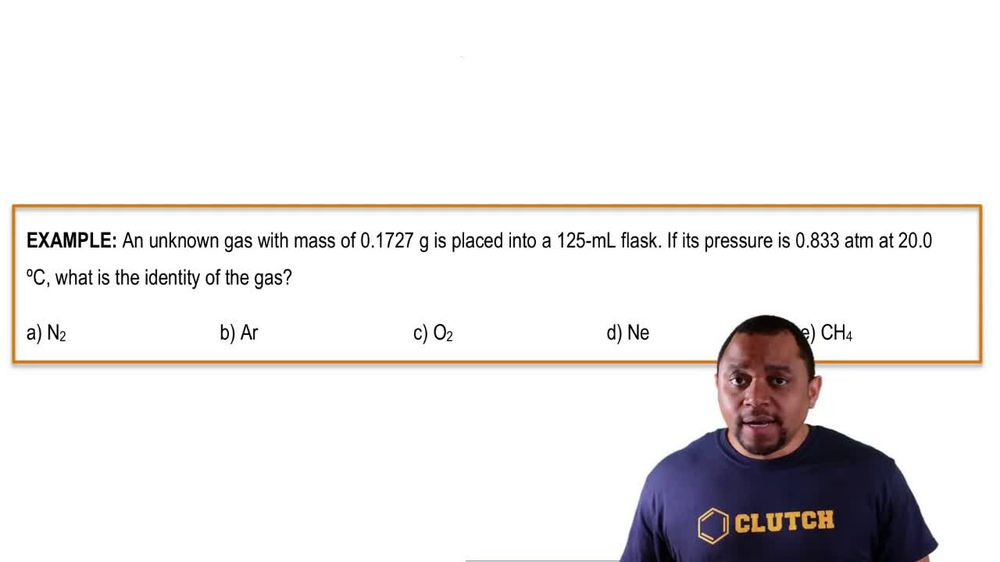Start typing, then use the up and down arrows to select an option from the list.
1. 7. Gases2. The Ideal Gas Law: Molar Mass# The Ideal Gas Law: Molar Mass Example 1

by Jules Bruno
164 views
1
1
1
here, we're told. Calculate the molar mass of a gas if 2.50 g occupies 0.995 leaders at 715 Tour and degrees Celsius. All right, we need to calculate Mueller Mass. Okay, Now more mass here equals grams over moles. So let's just say that grams over moles within the question they give us grams right off the bat. We have 250 g, but we don't have the moles. However, in the question, we see that we have volume. We have pressure and we have temperature. The P and T, we know are part of the ideal gas law. So we're gonna say PV equals NRT. With this information, we can isolate the moles of our gas so that by both sides here by rt and we're gonna say here that moles equals pressure. Times volume over rt. We need to convert our pressure into atmospheres. So remember, we have 715 tours and then we're going to stay here for everyone atmosphere that we have that's equal to 760 tours. Tours here would cancel out and we get as our pressure 0.9408 atmospheres, take those atmospheres and plug them in. So we have 9408 atmospheres. Our volume is already in leader, so we're good there. Remember, our is our gas constant, which is 0.8 to 06 leaders times atmospheres over moles, times K and temperature temperature has to be in Kelvin, so add to 73 15 to this. So when we do that, that's gonna give us 313. 15. Kelvin plug that in What units? Cancel out atmospheres. Cancel out leaders, Cancel out Kelvin's cancel out. And that's how we see. We'll have moles at the end. So here, when we do that, we get our moles as 0.364 moles take those moles and plug them into the Mueller mass formula. And doing that gives us ah value of 68.681 g per mole. Now here, 2.50 has three sig figs, three sig figs. Three sig figs, 40 has only one sigfig. So if we wanted to go by that one sigfig that will come out to 70 g per mole. It's not as accurate, but it's not a big deviation from our initial answer. So if we're going by significant figures, we can say that 70 g promote would be the more mass of our unknown gas.